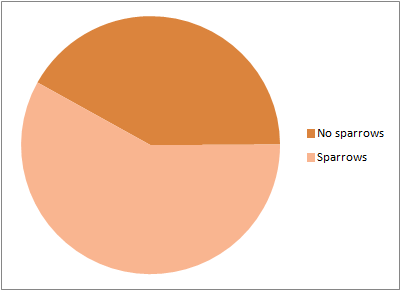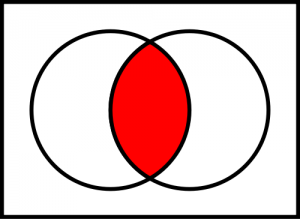# Google Search Q&A 4 – A beginner’s guide to set theory

According to our site stats, almost two thirds of visitors to our site are looking for information on sparrows. Don’t believe me? Here is a graph of the search strings that have found our site, grouped by whether or not they contain the word “sparrow”:See? It’s scientific fact. And 23 of those are variants on “is sparrow and dove same thing?” So, what does the mailbag have for us this time? (Please don’t be sparrows, please don’t be sparrows)

topless jamaica

red light district in frankfurt on map

rob liefeld wallpapers

… On second thoughts, let’s go with the sparrows.

sparrow in maths sets

Aww, who wants to learn some maths?

So, the maths you learn in school – arithmetic and algebra, mostly – represents only a very small part of modern mathematics. Numbers aren’t the only things used in maths; there are fields of maths where the objects being studied are directions, networks, knots, spaces, games, relationships, and mathematics itself, among other things.

One of the most important fields – the foundation of modern pure mathematics, in fact – is set theory, the study of objects which can contain other objects. For example, let’s create a new set, which we’ll call B. What is B? Why, it’s the set of all birds, of course!$B = \left \{ \text{house sparrow}, \text{hedge sparrow}, \text{rock dove}, \text{wood pigeon}, \text{european swallow}, \ \ldots \right \}$

(Did you know WordPress supports LaTeX typesetting? I DIDN’T! If the formulas don’t appear (so in other words, it looks like “\text{house sparrow}”), press F5)

So, “house sparrow” is a member of B. In shorthand (mathematicians are lazy people), we would write:$\text{house sparrow} \in B$

So, that’s a sparrow in a maths set! Ah, but the searcher wanted more than that. They wanted sparrow in maths sets, plural. Naive set theory places no restrictions on the sets we can create; any “well-defined” collection of objects we can name is a set!* So, let’s create another set! S, let’s say, is the set of birds called “sparrow”:$S = \left \{ \text{house sparrow}, \text{hedge sparrow}, \text{tree sparrow}, \text{rock sparrow},\ \ldots \right \}$

All sparrows are birds. This… should be common knowledge, I hope. In mathematical terms, the “set of all sparrows” is contained within “set of birds”, or for short:$S \subset B$

But, as we’ve already explained, not all birds called “sparrow” are true sparrows! So let’s be bird hipsters, and put the true sparrows into their own set, T:$T = \left \{ \text{house sparrow}, \text{tree sparrow}, \text{desert sparrow}, \text{Dead Sea sparrow},\ \ldots \right \}$

All true sparrows are called “sparrow”, but not all “sparrows” are true sparrows. So, again, we can write$T \subset S$

Now we can get a bit clever. All true sparrows are sparrows, but as we’ve already shown, all sparrows are themselves birds:$T \subset S \subset B$

So, all true sparrows are also birds!$T \subset B$

This useful property of set relationships is called transitivity, which simply means that you can collapse the relations down so that you skip the middle parts. Most of the familiar mathematical relations obey this – if a = b = c , then we immediately know that a = c – but there are plenty of every day relationships that are not transitive. Rock beats scissors, and scissors beats paper, but rock does not beat paper.

Anyway, now that we can relate sets to each other, let’s have a go at a bit of set maths! Don’t worry, it’s not as scary as it sounds.

We’ve got T, the set of all real sparrows. But what about the fake sparrows?! They’re important too! The definition of a fake sparrow is obvious – it’s any bird called “sparrow” that’s not a true sparrow. Intuitively, it’s easy to see how you’d get a list of fake sparrows – you’d take a list of birds called “sparrow”, and then take out all the true sparrows. With set theory, we can do just that, by subtracting T from S:$S \setminus T = \left \{ \text{hedge sparrow}, \text{rock sparrow}, \text{yellow-throated sparrow} \right \}$

Of these birds, the hedge sparrow is really an accentor, and the rock sparrow and yellow-throated sparrow are both petronias. The set of petronias, P, is:$P = \left \{ \text{rock sparrow}, \text{yellow-throated sparrow}, \text{yellow-spotted petronia}, \text{yellow-throated petronia}, \text{bush petronia} \right \}$Some of these petronias are also sparrows.** In other words, these birds are found in P, but they’re also found in S. The objects shared by two sets are its “intersection“.

The intersection is really easy to visualise – the picture on the right (conveniently in the public domain – thanks Wikipedia!) shows the intersection of two circles, as you’ll remember from all those Venn diagrams in school (they were teaching you set theory, and you didn’t even know it!

The intersection of S and P is written as:$S \cap P = \left \{ \text{rock sparrow}, \text{yellow-throated sparrow} \right \}$

We can also intersect P and T. We already know that no petronia is a true sparrow, so there are no birds common to both sets. This means that if we intersect them, we should get nothing. And so we do:$T \cap P = \varnothing$

The Ø symbol is the empty set, a set containing nothing at all. It’s the set theory equivalent of zero – any set subtracted from itself gives you the empty set.Finally, let’s put all the petronias and the sparrows together into one big friendly group of cute little birds (C, for “cute little bird”). To that, we use the “union” operation.

Union, illustrated on the right, gives you all objects that are in at least one set. Effectively, it’s like adding the two sets together, although it does make sure that you’ll never end up with two copies of the same object where the two sets overlap.

So, when we take the union of P and S, we get:$C = S \cup P = \left \{ \text{house sparrow}, \text{hedge sparrow}, \text{rock sparrow}, \text{bush petronia}, \text{yellow-spotted petronia},\ \ldots \right \}$

Aww, all those cute birds!

And, because the house sparrow is a cute little bird,$\text{house sparrow} \in C$

So, there you go. Sparrow in maths sets. I hope you’ve all learned something!

*This however leads to problems like Bertrand Russell’s famous paradox – suppose we create a set which contains all sets that do not contain themselves. Does this set contain itself as well? If it does, then it does not, and if it does not then it does. To get around this, mathematicians have created the much more rigorous Zermelo-Fraenkel set theory, which is far more complicated, but which contains lots of clever tricks to stop people creating self-referential sets. Mostly, this consists of a rule saying “You can’t make self-referential sets”.

** Yes, the “yellow-throated petronia” and “yellow-throated sparrow” are different birds, even though they’re both petronias and neither of them are true sparrows. TAXONOMY, EH?

This entry was posted in Birds, Google Searches, Maths and tagged , . Bookmark the permalink.

This site uses Akismet to reduce spam. Learn how your comment data is processed.